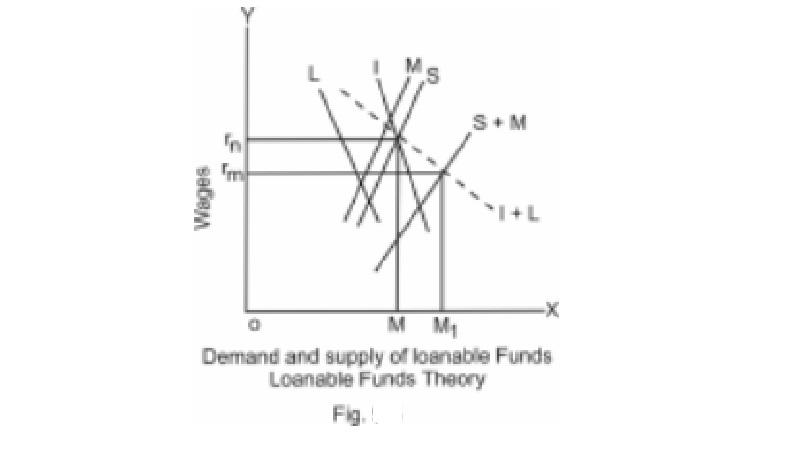Home | | Economics | | Economics | Loanable funds theory (Neo - classical theory) of Interest

Loanable funds theory (Neo - classical theory) of InterestThe loanable funds theory was developed by Knut Wicksell, Dennis Robertson and others. The loanable funds theory is wider in its scope than the classical theory of interest.

Loanable funds theory (Neo - classical theory) of Interest

The loanable funds theory was developed by Knut Wicksell, Dennis Robertson and others. The loanable funds theory is wider in its scope than the classical theory of interest. The term 'loanable funds' includes not only saving out of current income but also bank credit, dishoarding and disinvestments. But by saving, the classical economists referred only to saving out of current income. We know now that bank credit is an important source of funds for investment.

In the classical theory, saving was demanded only for investment. But according to loanable funds theory, the demand for funds arose, not only for investment but also for hoarding wealth.

The classical theory regarded interest as a function of saving and investment, (r = f (S.I.) But, according to loanable funds theory, the rate of interest is a function of four variables, i.e r = f (1,S M.L.) where r is the rate of interest, I = investment, S = saving, M = bank credit and L = desire to hoard or the desire for liquidity.

In Fig. The Curve 'S' represents savings, the curve 'M' represents bank credit (including dishoarded and disinvested wealth). The curve S + M represents total loanable funds at different rates of interest.

On the demand side, the curve I represents demand for investment. The curve L represents demand for idle cash balances or to hoard money. The curve I + L represents the total demand for loanable funds at different rates of interest. The market rate of interest rm is determined by the intersection of S + M curve and I + L curve. The aggregate demand for loanable funds is equal to the aggregate supply of loanable funds at this rate of interest. In the classical theory, rn which may be called the natural rate of interest is determined by the intersection of I and S curves. That is, when the rate of interest is rn, the demand for investment is equal to the supply of savings.

Criticism : There is no doubt that loanable funds theory is an improvement over the classical theory of interest. It has been criticized on the ground that it assumes that saving is a function of the rate of interest ; 2. it ignores the influence of the changes in the level of investment on employment, income and on savings.

Study Material, Lecturing Notes, Assignment, Reference, Wiki description explanation, brief detail

Related Topics